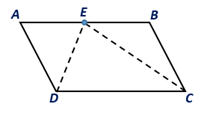Chapter 4.1, Problem 36E### Elementary Geometry for College St...

6th Edition
Daniel C. Alexander + 1 other
ISBN: 9781285195698

#### Solutions

Chapter
Section### Elementary Geometry for College St...

6th Edition
Daniel C. Alexander + 1 other
ISBN: 9781285195698
Textbook Problem
5 views

# When the bisectors of two consecutive angles of aparallelogram meet at a point on the remaining side,what type of triangle is: a) ∆DEC? b) ∆ADE? c) ∆BCE?To determine

a.

To find:

The type of the angle in ∆DECwhen the bisectors of two consecutive angles of the parallelogram ABCD meet at a point on the remaining side.

Explanation

Given:

The bisectors of two consecutive angles of the parallelogram ABCD are shown below.

Corollary:

A parallelogram is a quadrilateral in which both pairs of opposite sides are parallel.

In the given parallelogram ABCD.

 PROOF Statements Reasons 1. Parallelogram ABCD 1. Given 2. AD¯∥BC¯ 2. By the definition of a parallelogram 3. ∠EDC=∠D2 and∠DCE=∠C2 3. Since DE and CE are angle bisectors of ∠D and ∠C 4
To determine

b.

To find:

The type of the angle in ∆ADEwhen the bisectors of two consecutive angles of the parallelogram ABCD meet at a point on the remaining side.

To determine

c.

To find:

The type of the angle in ∆BCEwhen the bisectors of two consecutive angles of the parallelogram ABCD meet at a point on the remaining side.

### Still sussing out bartleby?

Check out a sample textbook solution.

See a sample solution

#### The Solution to Your Study Problems

Bartleby provides explanations to thousands of textbook problems written by our experts, many with advanced degrees!

Get Started

#### Find more solutions based on key concepts